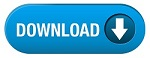NEW!  CBSE Papers PDF: Class-X, Class-XII

Disclaimer: This website is not at associated with CBSE, For official website of CBSE visit - www.cbse.nic.inQuestion paper
Exam Name :
INAO Olympiad Question Papers (Sr) 2008

## Part A: Multiple Choice Questions

1. The dimensions of Boltzmann constant (k) are
(a) M1L2T1
(b) M1L2 T−1
(c) M2L1T−1
(d) M1L2T−2
Note: Objections are raised over this question, because temperature dimensions are not specified. Thus, Question was removed from evaluation.

2. P. One can see absorption lines in the Solar spectrum.
Q. The core of the Sun has temperature of more than 1 million degree Celsius and the Solar surface has temperature of about 6000 degree Celsius.
Which of the following options is correct?
(a) Statement ‘P’ is correct but ‘Q’ is incorrect.
(b) Statement ‘P’ is incorrect but ‘Q’ is correct.
(c) Both the statements are correct and ‘Q’ is the correct reason of ‘P’.
(d) Both the statements are correct and ‘Q’ is not the reason of ‘P’.

3. When you stand on the ground, what is the distance of the horizon from you?
(a) 500 km
(b) 5 km
(c) 15 km
(d) 50 km

4. A regular barometer is thrown from the top of a building.If the barometer is freely falling, what will be the height of the mercury column?
(a) 100 cm
(b) 76 cm
(c) 50 cm
(d) 0 cm

5. P. Eclipses are not distributed evenly throughout the year, but happens only in certain months of a given year.
Q. Orbit of the Moon (around the Earth) makes an angle of roughly 5 degrees to the orbit of Earth (around Sun).
Which of the following options is correct?
(a) Statement ‘P’ is correct but ‘Q’ is incorrect.
(b) Statement ‘P’ is incorrect but ‘Q’ is correct.
(c) Both the statements are correct and ‘Q’ is the correct reason of ‘P’.
(d) Both the statements are correct and ‘Q’ is not the reason of ‘P’.

6. For the Earth, if the perihelion were 147 million km, approximately what will be the aphelion for the Earth?
Aphelion: Point farthest from the Sun in the orbit of a body about the Sun.
Perihelion: Point nearest from the Sun in the orbit of a body about the Sun.
(a) About 2 times the Perihelion, 300 million km
(b) About 3 times the Perihelion, 450 million km
(c) Slightly more than the perihelion, about 155 million km
(d) Exactly the same as the perihelion, 147 million km